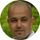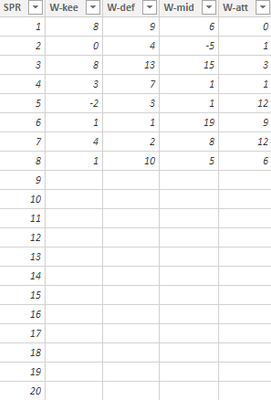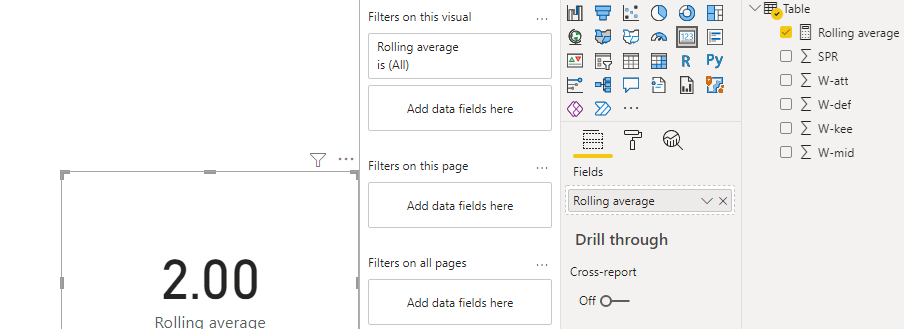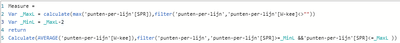cancel
Showing results for
Did you mean:Frequent Visitor

## Rolling average last 3 rows

Hi, I'm new here and I need some help.

I would like to calculate a "W-kee" rolling average over the last 3 rows in the table example as below:So the result should be ((1+4+1)/3=2), but I don't know what DAX formula I need to use.

I think I should use something based on nonblank rows for the W-kee column but I really have no glue.

1 ACCEPTED SOLUTIONCommunity Support

Hi,

According to your description, I can roughly understand your requirement, you can try this measure to get the expected result:

``````Rolling average =

var _lastrow=CALCULATE(MIN('Table'[SPR]),FILTER(ALL('Table'),[W-kee]=BLANK()&&[W-def]=BLANK()&&[W-mid]=BLANK()&&[W-att]=BLANK()))

return

CALCULATE(AVERAGE('Table'[W-kee]),FILTER(ALL('Table'),[SPR]<=_lastrow-1&&[SPR]>=_lastrow-3))``````

And you can get what you want, like this:Thank you very much!

Best Regards,

Community Support Team _Robert Qin

If this post helps, then please consider Accept it as the solution to help the other members find it more quickly.

9 REPLIES 9Community Support

Hi,

According to your description, I can roughly understand your requirement, you can try this measure to get the expected result:

``````Rolling average =

var _lastrow=CALCULATE(MIN('Table'[SPR]),FILTER(ALL('Table'),[W-kee]=BLANK()&&[W-def]=BLANK()&&[W-mid]=BLANK()&&[W-att]=BLANK()))

return

CALCULATE(AVERAGE('Table'[W-kee]),FILTER(ALL('Table'),[SPR]<=_lastrow-1&&[SPR]>=_lastrow-3))``````

And you can get what you want, like this:Thank you very much!

Best Regards,

Community Support Team _Robert Qin

If this post helps, then please consider Accept it as the solution to help the other members find it more quickly.Frequent Visitor

Great, that's the one I'm looking for.

Much appreciated!Super User

Hi @jdevalk ,

var Rolling Average= CALCULATE(Table[W-knee], FILTER(ALLSELECTED(Table[SPR]), ISONORAFTER( Table[SPR], MAX(Table[SPR]), asc)))
Return  (Rolling Average/ 3)

Mark this as a solution, if I answered your question. Kudos are always appreciated.

ThanksFrequent VisitorSuper User

Hi @jdevalk

Try this measure:

``````Measure=
Var _MaxL = calculate(max(table[SPR]),filter(table,table[W-kee]<>""))
Var _MinL = _MaxL-2
return
Calculate(avarage(table[W-kee]),filter(table,table[SPR]>=_MinL &&table[SPR]<=_MaxL ))``````

If this post helps, please consider accepting it as the solution to help the other members find it more quickly.Frequent VisitorSuper User

Hi @jdevalk

What is the format of [SPR] column?Frequent Visitor

Whole numberSuper User

Hi @jdevalk

Can you share your PBIX file with us (After removing sensetive data)?